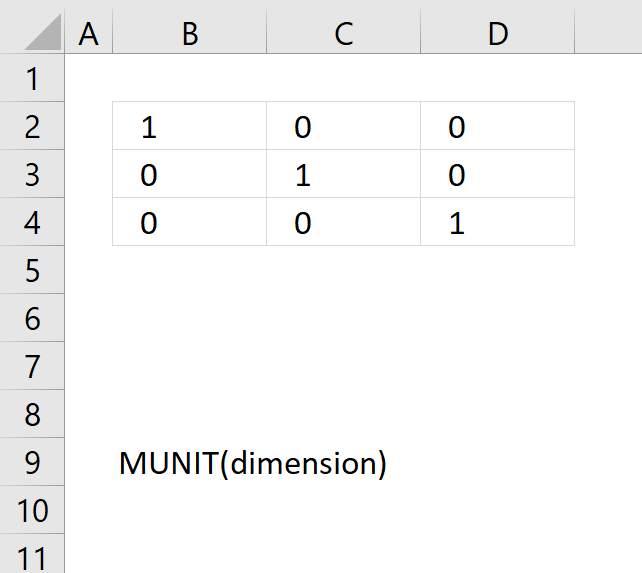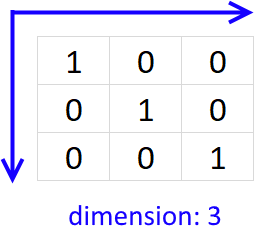Author: Oscar Cronquist Article last updated on May 05, 2022The MUNIT function calculates the identity matrix for a given dimension.

Array formula in cell B2:

=MUNIT(3)

The formula above returns an identity matrix with dimension 3, meaning the matrix is 3x3 and has ones on the main diagonal and zeros elsewhere.MUNIT(dimension)

### Arguments

 dimension Required. An integer that determines the dimension of the returning unit matrix.